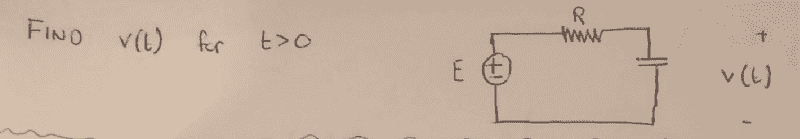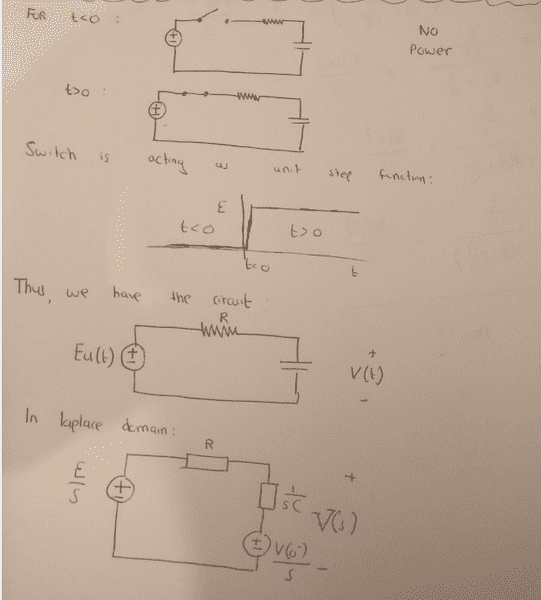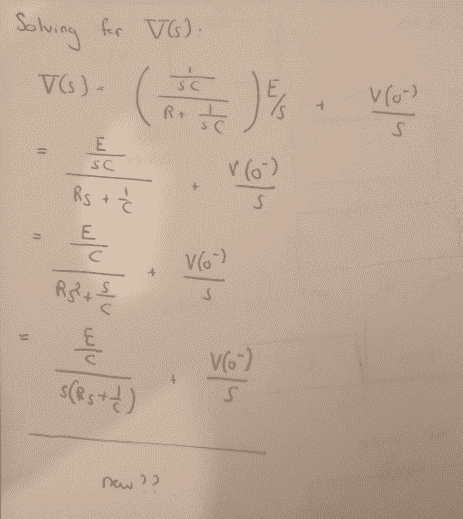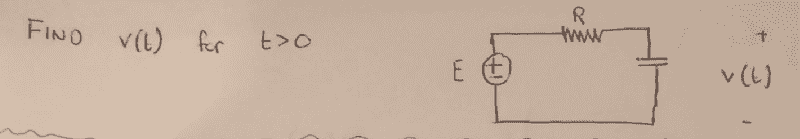# Laplace Transform on RC circuit

• Engineering
CoolDude420

## Homework Statement## The Attempt at a SolutionI'm kind of lost now, how do I go about getting this into the right form for partial fraction exapnsion. And also what do I do with the V(0'). There was no information given about it.

#### Attachments

magoo
What is the problem statement? It is not clear from what you supplied. Also, why do you have an initial charge on the capacitor when the switch was initially open?

CoolDude420The problem is to find v(t) for t>0. The diagram is a series combination of a voltage source E, resistor R and capcitance C. Where v(t) is the voltage across the capacitor. The step function diagram is for E not v(t)

#### Attachments

Staff Emeritus
Homework Helper
I'm kind of lost now, how do I go about getting this into the right form for partial fraction exapnsion.
Algebra.
And also what do I do with the V(0'). There was no information given about it.
It's reasonable to assume it's equal to 0, though the problem statement really should have included information about it.

CoolDude420
Algebra.

It's reasonable to assume it's equal to 0, though the problem statement really should have included information about it.

Any idea on what next "algebra" step to take?

Gold Member
take a look at any inverse laplace table. You need to find a way to get your system into one of those forms.
So...

E/C / [ S * (Rs+1/C) ] = A1 + [A2 / S] + [A3 / (Rs+1/C)]

Solve for A and B. How do you do that. Try setting s=0 and s= -1/(RC), which will cancel out the values